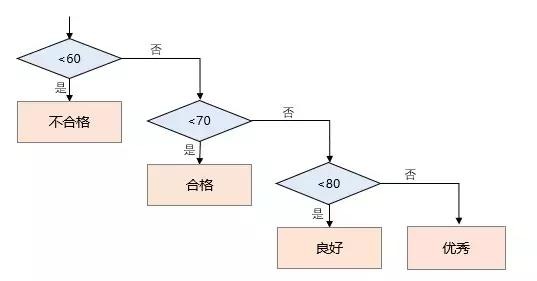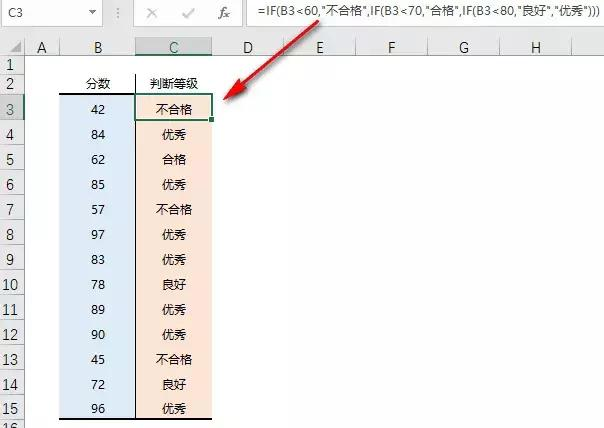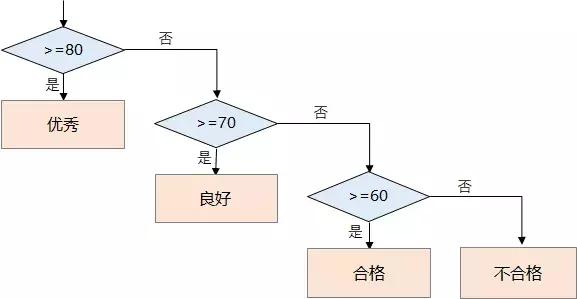IF 函数的语法如下：

=IF(条件判断,条件成立返回值,条件不成立返回值)

❶ 如果条件成立，返回一个值；

❷ 如果条件不成立，则返回另一个值。=IF(B3<60,"不合格",IF(B3<70,"合格",IF(B3<80,"良好","优秀")))=IF(B3>=80,"优秀",IF(B3>=70,"良好",IF(B3>=60,"合格","不合格")))